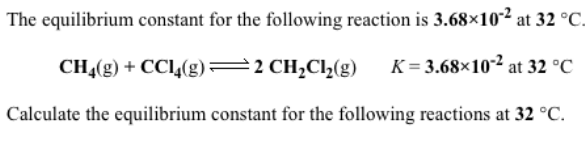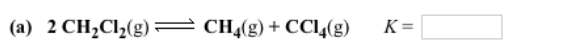# The equilibrium constant for the following reaction is 3.68x10^-2 at 32 degrees C. CH4(g) + CCl4(g) ⇌ 2 CH2Cl2(g) K=3.68x10^-2 at 32 degrees C. Calculate the equilibrium constant for the following reactions at 32 degrees C. (a) 2 CH2Cl2(g) ⇌ CH4(g) + CCl4(g)